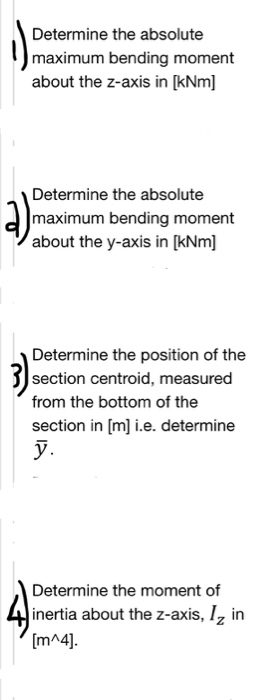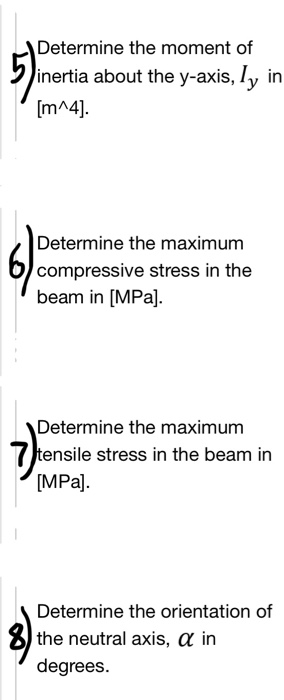# The simply supported beam is subjected to a uniform distributed load, w of 30 kN/m in...

###### Question:The simply supported beam is subjected to a uniform distributed load, w of 30 kN/m in the negative y-direction and a point load, P of 15 kN in the negative z-direction. The total length, L of the beam is 6 m. Answer the questions that follow: 'n Eenvoudige opgelegde balk word belas met 'n uniform verspreide belasting van w 30 kN/m in die negatiewe y-rigting en 'n puntlas P = 15 kN in die negatiewe z-rigting. Die totale lengte, L van die balk is 6 m. Beantwoord die vrae wat volg: L/2 P L2 250 mm t 12 mnm 250 mm 10 mmk t- 12 mt 200 mm
Determine the absolute maximum bending moment about the z-axis in [kNm] Determine the absolute maximum bending moment about the y-axis in [kNm] Determine the position of the section centroid, measured from the bottom of the section in [m i.e. determine Determine the moment of inertia about the z-axis, I, in [m^4].
Determine the moment of inertia about the y-axis, ly in Determine the maximum compressive stress in the beam in [MPa]. Determine the maximum tensile stress in the beam in MPa] Determine the orientation of the neutral axis, α in degrees.